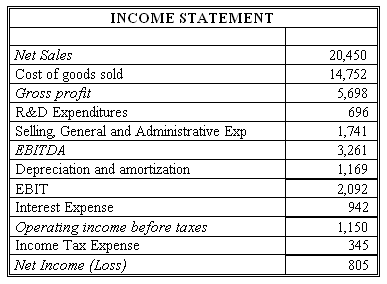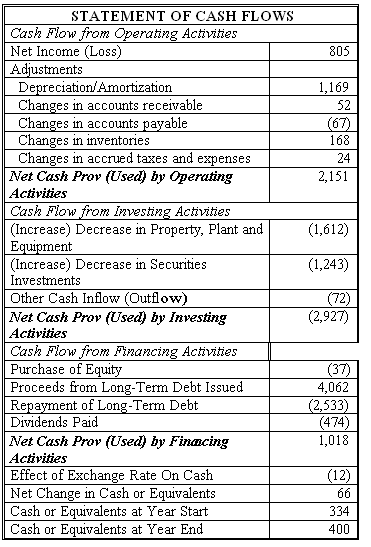### CFA Practice Question

There are 201 practice questions for this study session.

### CFA Practice Question

Connie's Sporting Goods (CSG) has net income of \$805 million for 2011. Using information from the CSG's financial statements below, use the EBITDA approach to find what FCFF and FCFE should be for CSG. Assume the income tax rate is 30%.Correct Answer: FCFF = EBITDA (1 - Tax rate) + Dep (Tax rate) - FCInv - WCInv
= 3261 (1- 0.3) + 1169 x 0.3 - 1612 - (-52 + 67 - 168 - 24) = \$1,198 million

FCFE = EBITDA (1 - Tax rate) + Dep (Tax rate) - Int (1 - Tax rate) - FCInv - WCInv + Net borrowing = 3261 (1 - 0.3) + 1169 (0.3) - 942 (1 - 0.3) - 1612 - (-52 + 67 - 168 - 24) + (4062 - 2533) = \$2,068 million